## How to do these STP gas and mass stoichiometry problems in general.

All of the problems in this set are stoichiometry problems with at
least one equation participant as a gas at STP. (a) Write and
balance the chemical equation. (2) Do the math in DA style using
1 mole gas at STP = 22.4 liters as a factor. In the following
problems ALL GASES ARE AT STP.

In this set of problems for the first time you will be
using the idea of stoichiometry. These problems always refer to a chemical
reaction. The chemical reaction must be first written and balanced. You will
be given an amount of one of the materials and be expected to find out how
much that corresponds to another one of the materials in that chemical reaction.
Because we are beginning with an amount of a material and we are looking for
another amount of (another) material, the DA method
is standardly used for this type of problem.

• Begin each problem with what you know, the
GIVEN material and the GIVEN amount in whatever units it is given.
• Change the GIVEN amount of material to units of
mols. In the case of gases at STP, the conversion factor is: 1 mol of gas =
22.4 liters at STP. In the case of masses, you must use the formula weight of
the material to change from mass to mols.
• Use the mol ratio to change from one material
to another. The mol ratio is the name (or symbol) of the material and the
coefficient of that material in the balanced chemical reaction.
• Change the mols of the new material to

PROBLEM #1, SET 2 – STP GAS AND MASS STOICHIOMETRY

1. How many moles of nitrogen gas is needed to react with 44.8
liters of hydrogen gas to produce ammonia gas?

3H2   +
N2
===>
2NH3

GIVEN: 44.8 L of H2 at STP.

FIND: mols of N2.

Here the sequence is: GIVEN liters of H2 at STP,
CHANGE liters of H2 at STP to mols of
H2, MOL RATIO to change from H2
to N2. There is no need to go any further
to change the N2 into mols, because the
mol ratio leaves the material in that unit anyway.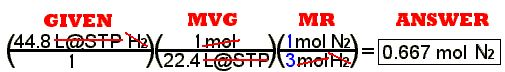The MVG is the “molar volume of gas at STP.” The
MR is the mol ratio. Notice the numbers in
blue in the mol ratio. Those numbers come from the
coefficients in the balanced chemical equation.

The math is:   44.8 ÷ 22.4 ÷ 3 =

PROBLEM #2, SET 2 – STP GAS AND MASS Stoichiometry

2. How many liters of ammonia are produced when 89.6 liters of
hydrogen are used in the above reaction?

The “above reaction” from problem #1 is: N2   +
3H2
===>
2NH3

GIVEN: 89.6 L of H2 at STP.

FIND: Volume of ammonia (in liters at STP)

Take the GIVEN quantity, use the Molar Volume of Gas at
STP (MVG) to change it to mols, change the material with the mol ratio (MR),
and change the mols of new material to the requested liters at STP using the
MVG again.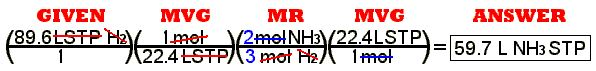The math is:   89.6 ÷ 22.4 ÷ 3 x 2 x 22.4 =
or, if the MVG’s cancel,   89.6 ÷ 3 x 2 =

PROBLEM #3, SET 2 – STP GAS AND MASS STOICHIOMETRY

3. Ten grams of calcium carbonate was produced when carbon
dioxide was added to lime water (calcium hydroxide in solution).
What volume of carbon dioxide at STP was needed?

CO2   +
Ca(OH)2
===>
CaCO3   +
H2O

GIVEN: 10.0 g = mass of calcium carbonate

FIND: Volume of carbon dioxide (in liters at STP)

Take the GIVEN quantity, a mass, use the Formula Weight of the given
quantity to change it to mols, change the material with the mol ratio (MR),
and change the mols of new material to the requested liters at STP using the
MVG.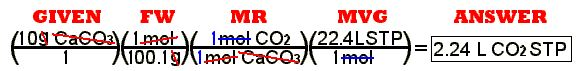The math is:   10 ÷ 100.1 x 22.4 =

PROBLEM #4, SET 2 – STP GAS AND MASS STOICHIOMETRY

4. When 11.2 liters of hydrogen gas is made by adding zinc to
sulfuric acid, what mass of zinc is needed?

Zn   +   H2
SO4
===>
H2   +   ZnSO4

GIVEN: 11.2 L of H2 at STP

FIND: mass of Zn

Take the GIVEN quantity, a volume at STP, use the MVG to change it to mols, change the material with the mol ratio (MR),
and change the mols of new material to the mass using the formula weight of the
new material. Find this pathway on the Stoichiometry RoadmapThe math is:   11.2 ÷ 22.4 x 65.4 =

PROBLEM #5, SET 2 – STP GAS AND MASS STOICHIOMETRY

5. What volume of ammonia at STP is needed to add to water to
produce 11 moles of ammonia water?

The balanced chemical equation is:
NH
3
+   H2
O
===>   NH4
OH

GIVEN: 11.0 mols of ammonia water (NH4OH)

FIND: Volume of ammonia gas (NH3) in liters at STP.

Take the GIVEN quantity, a number of mols, and directly use the mol ratio
(MR) to change to the other material. The mols of FIND material can be changed
to the requested liters at STP using the MVG.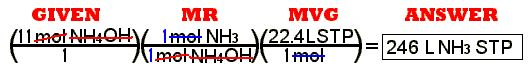The math is:   11 x 22.4 =

PROBLEM #6, SET 2 – STP GAS AND MASS STOICHIOMETRY

6. How many grams of carbonic acid is produced when 55 liters
of carbon dioxide is pressed into water?

There is no such thing as solid carbonic acid. It only exists in ionic form in
solution, but we can consider this exercise anyway.

The balanced chemical equation is:
CO
3
+   H2
O   ===>   H2
CO3

GIVEN: 55.0 mols of carbon dioxide (CO2)

FIND: mass of carbonic acid (H2CO3).

Take the GIVEN quantity, a number of mols, and directly use the mol ratio
(MR) to change to the other material. The mols of FIND material can be changed
to the requested mass the formula weight of the FIND material.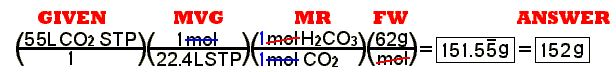The math is:   55 ÷ 22.4 x 62 =

PROBLEM #7, SET 2 – STP GAS AND MASS STOICHIOMETRY

7. magnesium hydroxide + ammonium sulfate ===> magnesium sulfate + water + ammonia

How much (grams) magnesium hydroxide do you need to use in the
above reaction to produce 500 liters of ammonia?

First change the word chemical equation to a balanced symbol equation:

Mg(OH)2
+ (NH4
)2
SO4 ===>
2H2
O + MgSO4
+ 2NH3

GIVEN: 500 liters of ammonia gas (NH3) at STP.

FIND: mass of magnesium hydroxide (Mg(OH)2).

Take the GIVEN quantity, a volume of gas at STP, and find the mols given with the
MVG, the molar volume of gas. Use the mol ratio (MR) to change to the other
material. The mols of FIND material can be changed to the requested mass the formula weight of the FIND material.
Find this pathway on the Stoichiometry Roadmap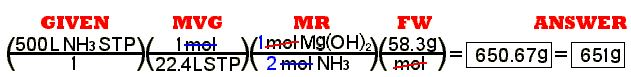The math is:   500 ÷ 22.4 ÷ 2 x 58.3 =

PROBLEM #8, SET 2 – STP GAS AND MASS STOICHIOMETRY

8. How much strontium bromide is needed to add to chlorine gas
to produce 75 liters of bromine gas?

GIVEN: 75 liters of bromine gas (Br2) at STP.

FIND: mass of strontium bromide (SrBr2).

Take the GIVEN quantity, a volume of gas at STP, and find the mols given with the
MVG, the molar volume of gas. Use the mol ratio (MR) to change to the other
material. The mols of FIND material can be changed to the requested mass the formula weight of the FIND material.
Find this pathway on the Stoichiometry RoadmapThe math is:   17 X 4 =

PROBLEM #8, SET 2 – STP GAS AND MASS STOICHIOMETRY

9. What mass of ammonium chlorate is needed to decompose to
give off 200 liters of oxygen?

10. Your car burns mostly octane, C8H18, as a fuel. How many
liters of oxygen is needed to burn a kilogram of octane?

11. copper + sulfuric acid ===> copper II sulfate

+ water + sulfur dioxide

How many moles of copper are needed to produce 1000 L of
SO2?

12. What volume of oxygen is needed to burn a pound of
magnesium?

13. How many grams of sodium do you have to put into water to
make 30 liters of hydrogen at STP?

14. ammonia gas and hydrogen chloride gas combine to make
ammonium chloride. What volume of ammonia at STP is needed to
react with 47.7 liters of hydrogen chloride at STP?

15. How many liters of oxygen are needed to burn 10 liters of
acetylene?